Next: About this document ... Up: lab_template Previous: lab_template

Subsections

# MA 1024 lab 1 - Curve Computations in the Plane

## Purpose

The purpose of this lab is to introduce you to curve computations using Maple for parametric curves and vector-valued functions in the plane.

## Getting Started

To assist you, there is a worksheet associated with this lab that contains examples and even solutions to some of the exercises. You can copy that worksheet to your home directory with the following command, which must be run in a terminal window, not in Maple.

cp ~bfarr/Curves2D_start.mws ~


You can copy the worksheet now, but you should read through the lab before you load it into Maple. Once you have read to the exercises, start up Maple, load the worksheet Vec2D_start.mws, and go through it carefully. Then you can start working on the exercises.

## Background

By parametric curve in the plane, we mean a pair of equations
and
for
in some interval
. A vector-valued function in the plane is a function
that associates a vector in the plane with each value ofin its domain. Such a vector valued function can always be written in component form as follows,
whereandare functions defined on some interval. From our definition of a parametric curve, it should be clear that you can always associate a parametric curve with a vector-valued function by just considering the curve traced out by the head of the vector.

For this lab, we will assume that we have a vector-valued functionthat gives the position at timeof a moving pointin the plane. The velocity of this point is given by the derivative
and the acceleration is given by the second derivative,
. In many applications of curvilinear motion, we need to know the magnitude of the velocity, or the speed. This is easy to compute - just take the magnitude. If you think of the speed as the rate of change of distance along the curve, and recall that arc length is distance measured along the curve, then you have the following interpretation of the speedwhere
is arc length.

If the speed is not zero for any value ofin the interval, then it is possible to define a unit vector,
that is tangent to the curve as follows.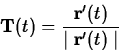Using this definition, you can write the velocity in the following form.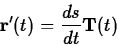This is not the most useful form for calculating the velocity, but it does lead to a useful way of thinking about the acceleration experience by a particle moving in a curvilinear path. If the path is a straight line, acceleration depends only on whether the particle is speeding up or slowing down. In a curve, however, there is an additional acceleration, called the centripetal acceleration, that is needed to keep the particle moving on the curve. The magnitude of this acceleration depends on the speed of the car and how much the path is curving. It turns out that you can quantify this with an intrinsic property of the curve called the curvature, usually denoted
, defined by the following equation.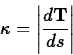That is, the curvature is the magnitude of the rate of change of the tangent vector
with respect to arc length. For example, the curvature of a straight line is zero and it can be shown that the curvature of a circle of radius
is the same for every point on the circle and is given by.

If the curvature is never zero for a particular curve, then we can define another intrinsic property of curve, the unit normal vectorby the following equation.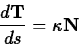It can be shown that at each point on the curve the vectordefined by this equation is a unit vector that is always perpendicular to the tangent vector
at that point. Furthermore, the unit normal vectoralways points in the direction of the centripetal acceleration required to keep a particle moving on the curve. In your text, the following important relation is derived.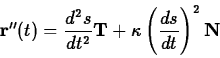To see why this equation is useful, recall that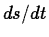is the speed, sois the rate of change of the speed. That is, this term measures whether the particle is speeding up or slowing down. Because this component of the acceleration is in the direction of the tangent vector it is often called the tangential acceleration, denoted by the symbol
. The component of the acceleration in the direction of the normal vector is called the normal acceleration, denoted
. In the case of motion on a circular path, the curvature is the reciprocal of the radius, so this term should be easily recognizable as the centripetal acceleration for uniform circular motion.

Computing these quantities is generally not an easy task. The Getting started worksheet for this lab describes commands from the CalcP package that simplify these calculations and provides examples for you to work from.

## Exercises

1. The vector valued functionrepresents uniform circular motion. That is, the particle moves at a constant speed around the unit circle in a counterclockwise fashion.
1. Use the ParamPlot command to visualize the motion for
and
for. What is the difference between the two values of
? You might want to use the Speed command to help you answer this question.
2. Compute the normal acceleration forand. Do your results agree with the formula from Physics that says that the centripetal acceleration is given by
?
2. For following vector function, compute the unit tangent vector, the unit normal vector, the curvature
, and the normal and tangential accelerations at the indicated value of. Include a plot of the curve for the giveninterval in your worksheet.
Compute,,,, andat
.
3. For the vector valued function in the previous exercise, plot the normal acceleration, the curvature, and the speed on the same graph. Can you explain why the maximum values of the curvature and the normal acceleration occur at different values of? You should be able to answer this question just by looking at the graph. You don't need to actually find the maximum values.Next: About this document ... Up: lab_template Previous: lab_template
William W. Farr
2003-01-13